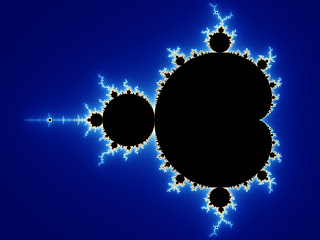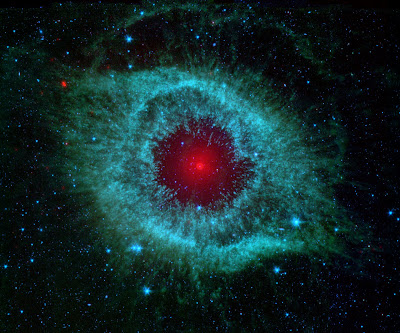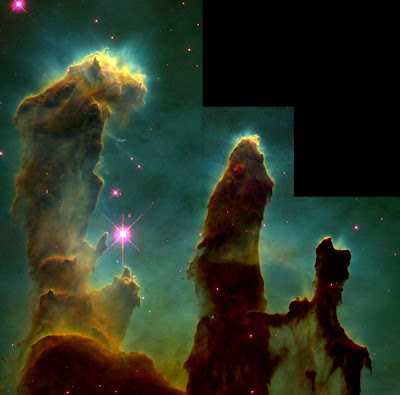## Saturday, January 31, 2009

### The Sun's Orbit and the Earth's Magnetic Field

Just as the Moon orbits the Earth, and the Earth orbits the Sun, the Sun also orbits the Milky Way galaxy. The Sun takes approximately 186 million years to orbit the Milky Way. Throughout the orbital period, the Sun intersects the arms of the galaxy. These arms are believed by some to be related to the reversal of the Earth's magnetic field. Yes, the Earth's magnetic field does reverse. It happens in periods of a anywhere from tens of thousands to millions of years. To put it in simple terms, a compass's handle would turn over (the arrow that faces north would face south, and the arrow that faces south would face north). A few thousand years before this happens, the magnetic field of Earth starts to weaken, and "holes" appear in it besides the usual ones at the poles. As this process goes on, Earth becomes more and more vulnerable to radiation and large meteors.

This being said, the activity of the magnetic field is also affected by the orbit of the Sun. When the Sun intersects the arm of our galaxy, the magnetic field is disturbed by the (relatively) close encounters with stars and other objects. This causes the magnetic field to be weak more than usual. During a gap between arms, there are little or no magnetic flips. Therefore, the field can be strong for millions of years in a row. This theory is reflected in Earth's history. For example, in the Cretaceous period, the Sun went through the longest empty gap in the galaxy. The magnetic field was quiet for nearly 40 million years. Then the magnetic field flipped five times in the next 20 million years. During this time, a 180 km meteor wiped out of 80% of life on Earth, including the dinosaurs. Also, a similar event occurred in the Permian period. After a 60 million year quiet zone, ten flips happened in the next ten million years. As the rapid flipping was occurring, a space rock more than 300 km across, one of the largest in history, destroyed over 95% of life on the planet. Note this this theory is not widely accepted at the current time.Map of the Milky Way.  The two events mentioned above are caused by the gap after the Outer Arm going clockwise.

If this theory is incorrect, another possible explanation is the dynamo theory. This theory is based on the fact that nearly everything (except some subatomic particles) have magnetic fields. This is due to the presence of electrons (up to the present, not much is known about where electrons get their charge, as this is one of the fundamentals of the very early Universe). Since the Earth has an iron core, a magnetic field is formed around that. But this is still not enough to explain the magnetic field around Earth, and this is here the dynamo theory comes in.

A combination of the convection on molten iron in the outer core and the Coriolis effect (the same force that allows low pressure systems to form, causing weather) creates powerful electric currents. Then, since electricity and magnetism are connected (see electromagnetism), another magnetic field is produced on top of the first one. With one field sustaining the other, a dynamo is formed. Other examples of dynamo in celestial bodies are conducting plasma in the Sun and other stars, and in active galactic nuclei (based on supermassive black holes). Disturbances in this magnetic field causes it to flip or reverse when the molten outer core's circulation is disrupted (e.g. a major asteroid impact, which gives a valid contradiction to the theory described earlier). The dynamo theory also explains the flipping of the solar magnetic field, which happens every 7 to 15 years, which is very often in contrast to the Earth's. The dynamo theory is the most widely believed at this point in time.

## Wednesday, January 28, 2009

### The Mandelbrot Set

Before reading this post, be sure you understand the previous post i: The Most Mysterious of All Numbers

One of the most famous examples of chaos in mathematics is the Mandelbrot Set. The Mandelbrot Set, discovered by Benoit Mandelbrot, has infinite complexity. But complexity is not necessarily rooted in complexity. The Mandelbrot Set is produced by by a method called iteration. Iteration is applying the same thing (or equation) over and over. The Mandelbrot Set is infinite iterations of a simple equation:

z → z^2+c

Where c is a complex number (as described in the post i: the most mysterious of all numbers). If c^2+c is continually applied than the first few terms in the sequence are:

0, c, c^2+c, (c^2+c)^2+c^2+c, (c^2+c)^2+c^2+c)^2+(c^2+c)^2+c^2+c

Every point on the complex plane is put into this equation, and if the result at each step escapes to infinity (grows larger and larger), than the point is left blank. If the steps of the sequence stay within a defined region and do not escape to infinity, the point is colored black. For example, the sequence for 1+i:

Step 1 0
Step 2 1+i
Step 3 (1+i)^2+(1+i)=1+2i-1+1+i=1+3i
.
.
.

As you can see, the sequence extends to infinity and is therefore colored white. Although the black regions and white regions are solid and uninteresting, the edges are filled with complex patterns.The "home" of the Mandelbrot Set.

For more images of the Mandelbrot Set, go to this site.

## Sunday, January 25, 2009

### i: The most mysterious of all numbers

The addition of pi to the number system made rationals not encompass all numbers. Therefore the system including both rational and irrational numbers is called the real numbers. Once the real numbers were discovered it seemed that they encompassed all numbers. But a closer look at square roots can change that.

Note: The equations below may be confusing. You do not need to fully understand the equations to understand complex numbers. Just try to understand the theory of it.

The square of a positive or a negative number is positive (the square of 0 is just 0). Therefore it seems that no number squares to a negative number, namely -1. Obviously, mathematicians had to come up with a new number for it. Therefore the quantity i was introduced. i was set to equal the square root of -1 forming the equation i^2=-1. i and other multiples of it make up the system called imaginary numbers. Also, the system encompassing all numbers is called the complex numbers. Complex numbers are in the form a+bi where a and b are ordinary real numbers. Make a=0 and you get bi, an imaginary number. Make b=0 and you get the constant a, a real number. In general, any square root of a negative number can be calculated by the simple equation (where square root of x=x^1/2) (-x)^1/2=i(x^1/2) where x is a positive number.

Complex numbers have other applications as well. For example, complex numbers provide solutions to any equation of the form

ax^0+bx^1+cx^2...+zx^n=0

In case the equation above confuses you, here are the basics: A complex number x can make the equations equal 0, and a, b, c, etc. are any numbers. Some of these equations couldn't previously be solved, which fills gaps that real numbers do not fill. The identity equation of this form is

x^2+1=0

This equation is solved by i (i^2=-1, -1+1=0, 0=0) which is the one of the identity complex numbers.

Before reaching the end of this post, one more topic must be covered: the complex plane. The complex plane is grid consisting of a real x-axis (a number line that increases left to right) and an imaginary y-axis (a number line that increases from down to up, except in multiples of i). Say you wanted to go to the point 3+2i. First, go three units right on the real x-axis, and then go two units up on the imaginary y-axis.

Today, phenomena on complex numbers are found in nature and used for physics as well as mathematics.

## Thursday, January 22, 2009

### The Fujiwhara Effect

When two hurricanes get too close to one another, a strange thing happens. The interaction is described by the Fujiwhara effect.
When two cyclones approach one another (get within a distance of about 1,450 miles) the two cyclone begin to orbit around a centroid. The centroid is located where the mass is balanced. The stronger a cyclone, the "heavier" it is. This situation isn't unlike Pluto and Charon, who orbit around a point just off Pluto's surface. Since Pluto is heavier, it dominates the interaction, and orbits closer to the center. Eventually one cyclone destroys the other, or, more rarely, they merge into one cyclone.

A few notable examples include an occurrence between Typhoon Pat and Tropical Storm Ruth in 1994. They completed one orbit around each other before merging into one cyclone. The most recent example in the Atlantic is Hurricane Wilma absorbing Tropical Storm Alpha in 2005.Two cyclones orbiting around each other in 1974.

## Monday, January 19, 2009

### The Coriolis Effect and the Pressure Gradient Force

How you ever wondered what enables weather on Earth? Well, it is the Coriolis effect. The Coriolis effect is a result of the Earth' rotation. To see it in action, draw a straight line on a spinning disc. The line you are drawing is straight, but it appears curved because the disc was moving. The same goes with the Earth. When a low-pressure system forms, the pressure-gradient force combines with the Coriolis effect. The pressure gradient force is the force that crates wind. Logically, air is pushed from the high-pressure to the low pressure area, just as air whooshes into a vacuum. This creates wind. The higher the difference in pressure and the shorter the distance, the stronger the wind is. Eventually, however, the Pressure Gradient Force is balanced off by the Coriolis effect, which sends the air in a spinning motion. In the northern hemisphere counterclockwise, in the Southern Hemisphere clockwise. For this reason, low-pressure systems cannot form over the Equator. If this spinning becomes associated with convection in tropical regions, the system becomes a tropical storm or hurricane.This is a polar low-pressure system over Iceland. It is NOT a tropical system.

## Friday, January 16, 2009

### Nebulae

A nebula is an cloud of gas that is the beginning stage in the life of a star (here). Nebulae form when interstellar medium clumps together and attracts more matter gravitationally. Eventually, gravity contracts the matter so much that that a infant star forms inside the nebula. At this point, the nebula is illuminated and therefore telescopes can spot it. Another way for a nebula to form is when a supernova explodes and leaves behind a cloud of gas, causing a chain reaction that leads to a nebula. Yet another type of nebula can form is from a smaller star such as our Sun. As the the Sun becomes a red giant, it lets off its outer layers, and these, later, can clump together, thereby forming a nebula. Nebulae can be large or small, depending on the amount of gas, and therefore the number of stars formed can vary as well.

Many types of nebulae exist, but most of them fall under the broader category of diffuse nebulae. The term diffuse nebula merely means that the nebula is irregular and has no exact edge or boundary. Diffuse nebulae are divided into subcategories based on how they are visible.

• Emission Nebula:  A nebula that is visible due to the ionized Hydrogen and Helium within it
• Reflection Nebula: A nebula that reflects the light of a nearby star
• Dark Nebula: A nebula that would normally be invisible, but is illuminated by a star behind it.

Nebulae are among the most beautiful objects in the Universe.The Helix Nebula.The famous Pillars of Creation seen in the Eagle Nebula.

## Tuesday, January 13, 2009

### Pi

π is the most famous irrational number known to mankind. It has been studied for over 3000 years and is one of the most important and most useful of mathematic entities. To sixty digits the value of π is...

3.1415926535897932384626433832795028841971693993751058209749445923078164...

I actually have the digits above memorized, a feat that took me awhile. If you like digits of π, here are some websites (here and here).

So, you might of heard π as 3.14 or 3.14159 but even 100, 1000, or even a million digits can't officially equal π. This is because π is an irrational number. An irrational number is a number that cannot be expressed as a ratio of two rational numbers. In simpler terms, an irrational number can't equal 3/7 or 231/576 or in general x/y, with x and y being rational numbers. Another example of an irrational number is the famed square root of 2 which equals approximately 1.414... Obviously 2π/2=π but π is irrational so the rule survives. Literally, π is the circumference of a circle whose diameter equals one. Therefore, the area and the circumference of any circle can be found by the formulae πr^2 and 2πr respectively (with r being the radius of the given circle). Some early methods of calculating π where by calculating the perimeter of polygons with n sides. As n increased the approximations would get more accurate because the more sides a polygon has the closer to a circle it will be. For example, in 480 A.D. Zu Chongzhi calculated an approximation of π with a polygon with an incredible 12288 sides!! This put π between 3.1415926 and 3.1415927, the most accurate approximation of pi for the next 900 years.

Today, more than a trillion (1,000,000,000,000) digits of π have be calculated using crazy formulae including infinite sums and factorials that extend to infinity.

## Saturday, January 10, 2009

### Plasma compared to other states of matter

Everyone knows about the main states of matter, solids, liquids, and gases. But fewer people know about the fourth state of matter: plasma. Plasma is similar to a gas but it is ionized. An ionized atom is one that has lost an electron to another atom. After losing an electron, an atom is positively charged, while the neighboring atom that gained the electron is negatively charged. Thus, plasma is affected by magnets. Plasma, although relatively unknown, is the most common state of matter in the Universe. It is the component of the interstellar medium, or the stuff that floats around in space. Plasma in even found naturally found on Earth. Believe it or not, lightning is made entirely of plasma. Superheated plasma (sometimes over 50,000 degrees Fahrenheit) strikes the ground or another cloud, creating the boom that we hear as thunder. Another common place where plasma is found naturally is polar aurorae (plural of aurora). These clouds of plasma are created by effect of the northern and southern magnetic poles.

Also, a theory of another type of plasma exists. It is called quark-gluon plasma (quarks and gluon are the smallest known building blocks of matter). It only exists at very high temperatures at which atoms, and not even protons and neutrons can exist.

## Wednesday, January 7, 2009

### Large Scale Universal Structure

Outside of our home Local Cluster of galaxies, structure and symmetry exist on a larger scale. All the super clusters near ours (within a few billion light-years) are connected by strands of galaxies called filaments. These filaments form hexagon shaped galactic structure, the inner part being mainly void. Outside of these structures however, no such symmetry exists. But, a large "wall" of galaxies marks the largest known Universal structure. It is called Sloan's Great wall and is 1,370,000,000 light-years long and approximately 1,000,000,000 light-years form Earth.Image showing filaments of galaxies creating structures such as superclusters and voids.This image shows structure at billions of light-years including Sloan's great wall and other major shapes.

Images provided by Wikipedia.

## Sunday, January 4, 2009

### Evolution of Galaxies

After galaxies and the first stars have formed, called Population III stars, rapid burning of Hydrogen fuel causes heavy elements, the first in the Universe in form. Then, when these stars die (most were massive enough to cause supernovas), heavier elements are dispersed into space, adding a slight amount of each to the next generation. Over the next two billion years, key structures are seen in the galaxy (see Types of Galaxies) and as more and more supernovas occur, the abundance of heavy elements slowly increases, eventually allowing the formation of solar systems. Then, as time goes on, formation of new stars slows. Sometimes, especially near the centers of galaxy clusters, two galaxies merge. This is how an elliptical galaxy forms.This is a photo of two merging galaxies, collectively called NGC 4676.
Image provided by Wikipedia.

## Thursday, January 1, 2009

### Types of Galaxies

Galaxies are separated into three main types. These types are elliptical, spiral, and barred spiral. Elliptical galaxies, range from perfectly spherical to flattened discs. About 12% of the galaxies in the Universe are elliptical, and they can hold from hundred millions to trillions of stars and are commonly found at the centers of star clusters. Elliptical galaxies are older and very little star formation occurs in them, resulting in an old population of stars.The next type, spiral galaxies, are centers of ongoing star formation, and are found in low density areas of galaxy clusters. Young and luminous stars illuminate bands in the galaxy, giving it a spiral appearance, but in reality, there are unseen stars outside the bands, forming what is called a galaxy halo.These galaxies are almost identical to barred spiral galaxies and 2/3 of all spirals have bars. The structure is thought to help stellar formation.Also, a quarter of all galaxies are irregular. They were formerly ellipticals or spirals, but were deformed by gravitational pull.Images provided by Wikipedia.